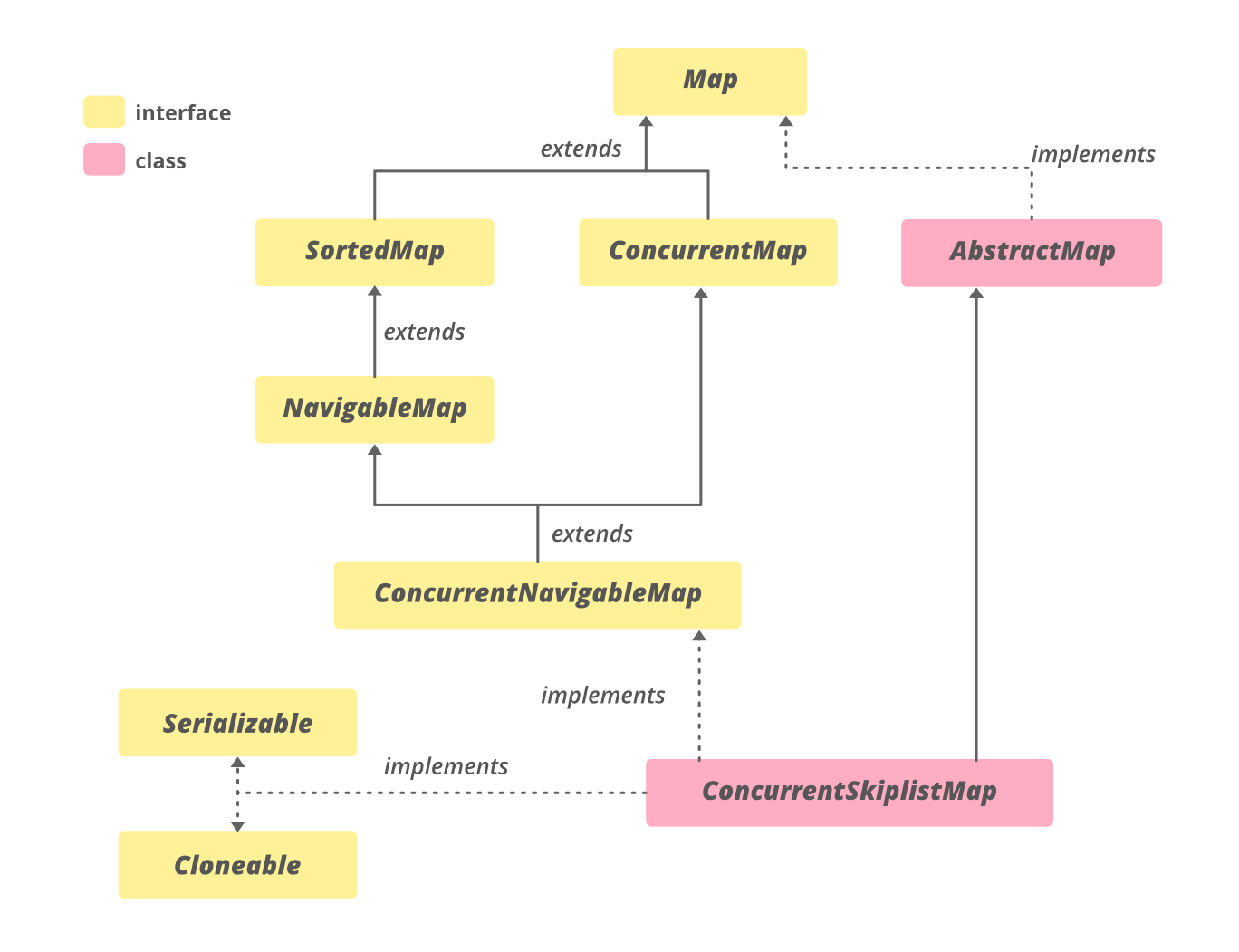# ConcurrentSkipListMap in Java with Examples

• Difficulty Level : Expert
• Last Updated : 24 Nov, 2020

The ConcurrentSkipListMap class is a member of the Java Collections Framework. It was introduced in JDK 1.6, it belongs to java.util.concurrent package. The ConcurrentSkipListMap is a scalable implementation of ConcurrentNavigableMap. All the elements are sorted based on natural ordering or by the Comparator passed during it’s construction time. This class uses a concurrent variation of SkipList data structure providing log(n) time cost for insertion, removal, update, and access operations. These operations are safe for executing concurrently by multiple threads.

Declaration

public class ConcurrentSkipListMap<K,​V> extends AbstractMap<K,​V> implements ConcurrentNavigableMap<K,​V>, Cloneable, Serializable

Here, K is the key Object type and V is the value Object type.

The Hierarchy of ConcurrentSkipListMapIt implements Serializable, Cloneable, ConcurrentMap<K,​V>, ConcurrentNavigableMap<K,​V>, Map<K,​V>, NavigableMap<K,​V>, SortedMap<K,​V> interfaces and extends AbstractMap<K, V> class.

### Constructors of ConcurrentSkipListMap

1. ConcurrentSkipListMap(): Constructs a new, empty map, sorted according to the natural ordering of the keys.

ConcurrentSkipListMap<K, V> cslm = new ConcurrentSkipListMap<K, V>();

2. ConcurrentSkipListMap​(Comparator<? super K> comparator): Constructs a new, empty map, sorted according to the specified comparator.

ConcurrentSkipListMap<K, V> cslm = new ConcurrentSkipListMap​<K, V>(Comparator<? super K> comparator);

3. ConcurrentSkipListMap​(Map<? extends K,​? extends V> m): Constructs a new map containing the same mappings as the given map, sorted according to the natural ordering of the keys.​

ConcurrentSkipListMap<K, V> cslm = new ConcurrentSkipListMap<K, V>​(Map<? extends K,​? extends V> m);

4. ConcurrentSkipListMap​(SortedMap<K,​? extends V> m): Constructs a new map containing the same mappings and using the same ordering as the specified sorted map.

ConcurrentSkipListMap​<K, V> cslm = new ConcurrentSkipListMap​<K, V>(SortedMap<K,​? extends V> m);

Example

## Java

 `// Java Program to Demonstrate``// ConcurrentSkipListMap`` ` `import` `java.io.*;``import` `java.util.*;``import` `java.util.concurrent.*;`` ` `class` `ConcurrentSkipListMapExample {``    ``public` `static` `void` `main(String[] args)``    ``{``        ``// create an instance of ConcurrentSkipListMap``        ``ConcurrentSkipListMap cslm``            ``= ``new` `ConcurrentSkipListMap();`` ` `        ``// Add mappings using put method``        ``cslm.put(``"3"``, ``"Geeks"``);``        ``cslm.put(``"2"``, ``"from"``);``        ``cslm.put(``"1"``, ``"Hi!"``);``        ``cslm.put(``"5"``, ``"Geeks"``);``        ``cslm.put(``"4"``, ``"for"``);`` ` `        ``// print to the console``        ``System.out.println(``"Initial Map : "` `+ cslm);`` ` `        ``// print key-value pair whose key is greater than 2``        ``System.out.println(``"ceilingEntry-2: "``                           ``+ cslm.ceilingEntry(``"2"``));`` ` `        ``// get the descending key set``        ``NavigableSet navigableSet = cslm.descendingKeySet();`` ` `        ``System.out.println(``"descendingKeySet: "``);`` ` `        ``// Iterate through the keySet``        ``Iterator itr = navigableSet.iterator();``        ``while` `(itr.hasNext()) {``            ``String s = (String)itr.next();``            ``System.out.println(s);``        ``}`` ` `        ``// print the first mapping``        ``System.out.println(``"firstEntry: "``                           ``+ cslm.firstEntry());`` ` `        ``// print the last mapping``        ``System.out.println(``"lastEntry: "``                           ``+ cslm.lastEntry());`` ` `        ``// remove the first mapping and print it``        ``System.out.println(``"pollFirstEntry: "``                           ``+ cslm.pollFirstEntry());`` ` `        ``// print the first mapping``        ``System.out.println(``"now firstEntry: "``                           ``+ cslm.firstEntry());`` ` `        ``// remove the last mapping and print it``        ``System.out.println(``"pollLastEntry: "``                           ``+ cslm.pollLastEntry());`` ` `        ``// print the last mapping``        ``System.out.println(``"now lastEntry: "``                           ``+ cslm.lastEntry());``    ``}``}`

Output

```Initial Map : {1=Hi!, 2=from, 3=Geeks, 4=for, 5=Geeks}
ceilingEntry-2: 2=from
descendingKeySet:
5
4
3
2
1
firstEntry: 1=Hi!
lastEntry: 5=Geeks
pollFirstEntry: 1=Hi!
now firstEntry: 2=from
pollLastEntry: 5=Geeks
now lastEntry: 4=for```

### Basic Operations

The put() method of ConcurrentSkipListMap associates the specified value with the specified key in this map. If the map previously contained a mapping for the key, the old value is replaced.

## Java

 `// Java Program to demonstrate adding``// mappings to a ConcurrentSkipListMap`` ` `import` `java.util.concurrent.*;`` ` `class` `AddingMappingsExample {``    ``public` `static` `void` `main(String[] args)``    ``{`` ` `        ``// Initializing the map``        ``ConcurrentSkipListMap cslm``            ``= ``new` `ConcurrentSkipListMap();`` ` `        ``// Adding elements to this map``        ``for` `(``int` `i = ``1``; i <= ``9``; i++)``            ``cslm.put(i, i);`` ` `        ``// put() operation on the map``        ``System.out.println(``"After put(): "` `+ cslm);``    ``}``}`

Output

`After put(): {1=1, 2=2, 3=3, 4=4, 5=5, 6=6, 7=7, 8=8, 9=9}`

2. Remove Mappings

The remove() method of ConcurrentSkipListMap removes the mapping for the specified key from this map. The method returns null if there is no mapping for of that particular key. After this method is performed the size of the map is reduced. To remove the first entry and last entry of the map, we can use pollFirstEntry() and pollLastEntry() respectively.

## Java

 `// Java Program Demonstrate removing``// mappings from ConcurrentSkipListMap`` ` `import` `java.util.concurrent.*;`` ` `class` `RemovingMappingsExample {``    ``public` `static` `void` `main(String[] args)``    ``{`` ` `        ``// Initializing the map``        ``ConcurrentSkipListMap cslm``            ``= ``new` `ConcurrentSkipListMap();`` ` `        ``// Adding elements to this map``        ``for` `(``int` `i = ``1``; i <= ``6``; i++)``            ``cslm.put(i, i);`` ` `        ``// remove() operation on the map``        ``cslm.remove(``5``);`` ` `        ``// print the modified map``        ``System.out.println(``"After remove(): "` `+ cslm);`` ` `        ``// remove the first mapping and print it``        ``System.out.println(``"pollFirstEntry: "``                           ``+ cslm.pollFirstEntry());`` ` `        ``// remove the last mapping and print it``        ``System.out.println(``"pollLastEntry: "``                           ``+ cslm.pollLastEntry());``           ` `          ``// Print final map``          ``System.out.println(``"map contents: "` `+ cslm);``    ``}``}`

Output

```After remove(): {1=1, 2=2, 3=3, 4=4, 6=6}
pollFirstEntry: 1=1
pollLastEntry: 6=6
map contents: {2=2, 3=3, 4=4}```

3. Iterating

We can use the Iterator interface to traverse over any structure of the Collection Framework. Since Iterators work with one type of data we use Entry< ? , ? > to resolve the two separate types into a compatible format. Then using the next() method we print the elements of the ConcurrentSkipListMap.

## Java

 `// Java Program to demonstrate iterating``// over ConcurrentSkipListMap`` ` `import` `java.util.concurrent.*;``import` `java.util.*;`` ` `class` `IteratingExample {``    ``public` `static` `void` `main(String[] args)``    ``{``        ``// create an instance of ConcurrentSkipListMap``        ``ConcurrentSkipListMap cslm``            ``= ``new` `ConcurrentSkipListMap<>();`` ` `        ``// Add mappings using put method``        ``for` `(``int` `i = ``0``; i < ``6``; i++) {``            ``cslm.put(i, i);``        ``}`` ` `        ``// Create an Iterator over the``        ``// ConcurrentSkipListMap``        ``Iterator > itr``            ``= cslm.entrySet().iterator();`` ` `        ``// The hasNext() method is used to check if there is``        ``// a next element The next() method is used to``        ``// retrieve the next element``        ``while` `(itr.hasNext()) {``            ``ConcurrentSkipListMap``                ``.Entry entry``                ``= itr.next();``            ``System.out.println(``"Key = "` `+ entry.getKey()``                               ``+ ``", Value = "``                               ``+ entry.getValue());``        ``}``    ``}``}`

Output

```Key = 0, Value = 0
Key = 1, Value = 1
Key = 2, Value = 2
Key = 3, Value = 3
Key = 4, Value = 4
Key = 5, Value = 5```

### Methods declared in interface java.util.SortedMap

My Personal Notes arrow_drop_up